# How to quantify time-variability of non-periodic sources?¶

In our last few G+ hangouts we discussed periodic sources in great depth. Luisa has compared at least three different period finding methods and I checked her results with the implementation that Katja and I use in our python code and find good agreement. So, while there are a few issues to decide (and that might change from cluster to cluster) including:

• What is the maximum length of the period that we believe, i.e. does the period fit at least 2, 3 or 4 times into the length of the monitoring?
• What do we use as cut-off for the significance? Luisa uses a FAP of 5%, which seems OK to me.

I think, we basically have this solved. However, only the minority of all sources are strictly periodic during the observations. All other indicators that we talked about so far, do not take into account the shape of the lightcurve. They only characterize the set of magnitudes as an ensemble, e.g. the mean, max, min, standard deviation etc. don't care about the time ordering. Even the Stetson index picks out correlated variability, but is agnostic about the time ordering. If IRAC1 and IRAC2 lightcurves are reordered together, the Stetson index will stay the same (Each measurement point has [m_36, m_45, time], but stetson only uses [m_36, m_45]).

In her code Maria uses the autocorrelation function to describe the typical time scale in a lightcurve. Here, I want to check if her definition of parameters is useful for the cluster I work on (L1688). Since its short cadence data is comparable to the set-up of the other clusters, that can hopefully serve as a guide for all cluster papers.

This is a so-called "ipython notebook". While you probably see it rendered as static html, you can download the .ipynb and rerun and change the code on your computer if you want and (that's my main motivation for choosing this format) I can go back myself, read the text I wrote to understand what I did, make changes to the code and see the new plots. If you don't care about the python code, just ignore those lines and look at plots and my ramblings in text only.

In :
%pylab inline

Welcome to pylab, a matplotlib-based Python environment [backend: module://IPython.zmq.pylab.backend_inline].

In :
import sys
import numpy as np
import scipy.signal

import numpy as np

sys.path.append('/data/hguenther/Dropbox/code/python/YSOVAR')
from YSOVAR import atlas
import YSOVAR.lightcurves as lc

In :
inroot = '/data/hguenther/misc/YSOVAR/RhoOph/'
data = atlas.dict_from_csv(inroot + 'YSOVAR859.csv', match_dist = 0.)

Reading csv file - This may take a few minutes...
Processing dict for source 0 of 1909
Processing dict for source 100 of 1909
Processing dict for source 200 of 1909
Processing dict for source 300 of 1909
Processing dict for source 400 of 1909
Processing dict for source 500 of 1909
Processing dict for source 600 of 1909
Processing dict for source 700 of 1909
Processing dict for source 800 of 1909
Processing dict for source 900 of 1909
Processing dict for source 1000 of 1909
Processing dict for source 1100 of 1909
Processing dict for source 1200 of 1909
Processing dict for source 1300 of 1909
Processing dict for source 1400 of 1909
Processing dict for source 1500 of 1909
Processing dict for source 1600 of 1909
Processing dict for source 1700 of 1909
Processing dict for source 1800 of 1909
Processing dict for source 1900 of 1909
Cleaning up dictionaries
Floor values  {'IRAC2': 0.007, 'IRAC1': 0.01}


### Get an exemplary lightcurve¶

data now holds all lightcurves for this one cluster. However, it's probably good enough to check out most things on the single example. Let's extract one lightcurve and put in variables with really short names (t and m), because I'll type it often.

In :
t = data['t36']
m = data['m36']
print 'number of datapoints in this lightcurve ', len(t)

number of datapoints in this lightcurve  105

In :
fig = plt.figure(figsize = (15,4))
ax.plot(t,m,'rs')
ax.invert_yaxis()
ax.set_xlabel('Time [MJD]')
ax.set_ylabel('[3.6]')

Out:
<matplotlib.text.Text at 0x1447f910>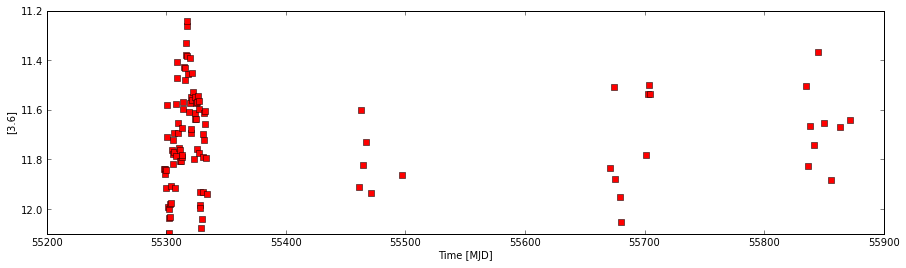Or, here is a zoom of the first short cadence monitoring:

In :
plt.plot(t,m,'rs')
plt.plot(t,m,'r')
plt.xlim([55290, 55340])
ax = plt.gca()
ax.invert_yaxis()
ax.set_xlabel('Time [MJD]')
ax.set_ylabel('[3.6]')

Out:
<matplotlib.text.Text at 0x14498310>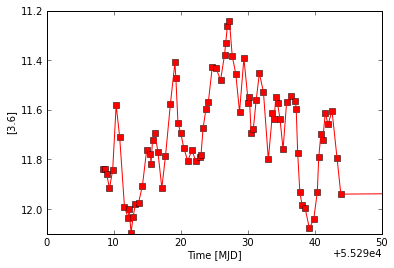### Check that our exemplary lightcurve is not periodic¶

In :
indq1 = t < 55400
from YSOVAR.lombscargle import fasper
test = fasper(t[indq1], m[indq1], 4,1)
print 'FAP: ', test, 'period: ',1./test[test]

FAP:  3.18195743107e-07 period:  141.8233904

In :
plt.plot(test, test)
plt.xlabel('fequency [1/days]')
plt.ylabel('Spectral power')

Out:
<matplotlib.text.Text at 0x4407b90>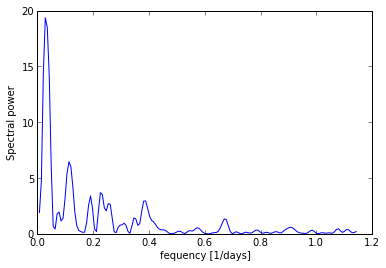So, the lightcurve we picked as example has 105 points, which means it's one of the best I have in my cluster. There is no periodicity that I believe. The LS periodogram of the short cadence data (only the first block of the observation) picks up something "significant" with a period of 142 days - much longer than the monitoring block. Clearly, this is indicative of a long term trend, but not of a real period. However, there are other peaks in the periodogram, that might indicate some sort of preferred time scale and the plot of the lightcurve shows visually that the distribution is not totally random. There is certainly some correlated structure to it. The question is how to find it.

### Time sampling of the lightcurve¶

In :
plt.hist(np.diff(t), range = [0,2])
plt.xlabel('$\\delta$ t [days] between two datapoints')
plt.title('Histogram of $\\delta$ t')

Out:
<matplotlib.text.Text at 0xdbf3ad0>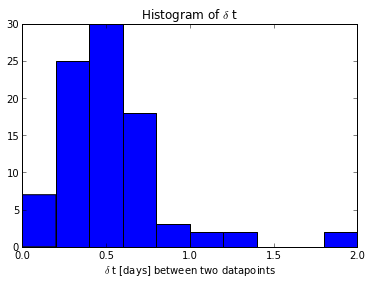Clearly, the time sampling of this lightcurve is fairly uneven, this difference between two samples is typically less than one day. There is some pattern to it as well:

In :
plt.plot(np.diff(t), 'bs')
plt.plot(np.diff(t), 'b')
plt.xlabel('number of time step')
plt.ylabel('time step between datapoints [days]')
plt.xlim([0, 75])
plt.ylim([0,1])

Out:
(0, 1)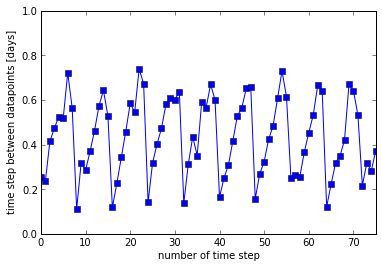So, the smallest time step is 0.1 days, the largest (in the first epoch) is 0.8 days. Thus, if I want to a straightforward autocorrelation function in the same way that Maria does, then I would need to resample the lightcurve on some regular grid. What grid size should I chose? Probably 0.1 days, otherwise I loose information, but that mean I need to "make up" (interpolate) in some way between the datapoints that are further apart. That could clearly introduce new correlations.

So, let me first see how far I can get without interpolating the lightcurve.

## Autocorrelation of an unevenly sampled lightcurve¶

First, I normalize the lightcurve to mean = 0 and stddev = 1, then I calculate for all possible pairings of datapoints $i$ and $j$ the time difference $\delta t_{ij}$ and $\frac{(m_i - \hat m) * (m_j- \hat m)}{\sigma_m^2}\$, where $\hat m\$ is the mean of all $m$ and $\sigma_m$ is the standard deviation. The resulting point cloud is plotted below. Now, instead of binning the original lightcurve, I bin this point cloud using different bin widths (colored lines in the plot). The maximum $\delta t$ is about 600 days but I zoom into the plot to show the interesting stuff for small time scales.

This binned version is exactly equal to the autocorrelation function (ACF) in the limit a a regular time sampling. (Contact me if you want to see the math.)

In :
m_norm = lc.normalize(m)
dt, dm = lc.delta_corr_points(t, m_norm, m_norm)

In :
plt.plot(dt, dm, 'y.')
for scale in [0.1, 0.5, 1.0]:
timebins = np.arange(0, np.max(dt), scale)
autocorr, n_in_bin = lc.slotting(timebins, dt, dm)
plt.plot(timebins, autocorr, lw = 2., label = 'bin width = ' + str(scale))
plt.xlim([0,10])
plt.ylim([-1,2])
plt.xlabel('time scale [days]')
plt.legend(loc = 'upper right')

Out:
<matplotlib.legend.Legend at 0x1e66ef90>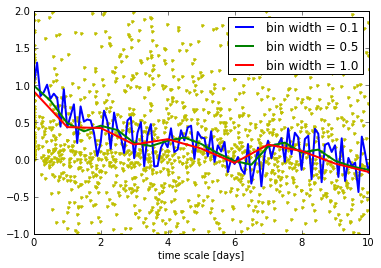On a plot like this, Maria defines three numbers:

• The coherence time, where the autocorrelation function falls below 0.5 for the first time.
• The timescale as the position of the first positive peak (the first peak after the function went below 0).
• The autocorrelation value, the height of the first positive peak.

Looking at the plot, we can see that these numbers are not very good here. The green line (0.1 day binning) is clearly too noisy and for the 0.5 and 1.0 binning, e.g. the coherence time is 1.0. That's not very useful, if the bin size is 1.0 already. Similarly for the first peak, which is formally around 7 days. It's not clear if that is any better than the peak at 4.5 days, because for some binning the function drops below 0 already at 3.5 days, for others (0.5 and 1.0 days) is does not.

So, I need to find some more robust measure for this.

### Comparing with the autocorrelation function for random noise¶

For random, gaussian noise the autocorrelation function $ACF\$ is

$ACF(0) = 1$

$ACF(x \ne 0) = N(-\frac{1}{n}, \frac{1}{n})$

where $n$ is the number of elements and $N(\mu, \sigma^2)\$ is a normal distribution with mean $\mu$ and standard diviation $\sigma$ (see, e.g. Feigelson & Babu, Modern Statistical Methods for Astronomy). So, I'll overplot the 3 $\sigma$ limits on the plot.

In :
tq1 = t[t < 55400]
plt.plot(dt, dm, '.y')
for scale in [0.1, 0.5, 1.0]:
timebins = np.arange(0, np.max(dt), scale)
autocorr, n_in_bin = lc.slotting(timebins, dt, dm)
plt.plot(timebins, autocorr, lw = 2., label = 'bin width = ' + str(scale))
plt.xlim([0,10])
plt.ylim([-1,2])
plt.xlabel('time scale [days]')
plt.barh(-(1./len(tq1) + 3./np.sqrt(len(tq1))), 10., height = 2. * 3 / np.sqrt(len(tq1)), color = 'k', label='$3\\sigma$ interval for white noise', alpha=0.4)
plt.legend(loc = 'upper right')

Out:
<matplotlib.legend.Legend at 0x9cee2d0>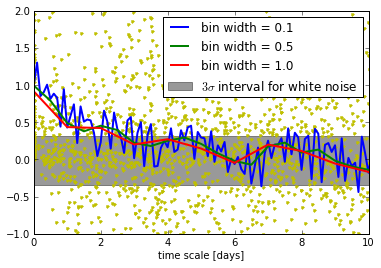In :
def slot_n(x, y, n = 11):
ind = np.argsort(x)
x = x[ind]
y = y[ind]
n_bins = len(x) / int(n)
x = np.reshape(x[:n_bins * n], (-1,n))
y = np.reshape(y[:n_bins * n], (-1,n))
return x.mean(axis=1), y.mean(axis=1), np.std(y, axis=1)

plt.plot(dt, dm, 'y.')
for n in :
timebins, autocorr, std_autocorr = slot_n(dt, dm, n=n)
r = autocorr
z = 0.5 * np.log((1. + r) / (1. - r))
plt.plot(timebins, autocorr, lw = 2., label = 'binned ACF')
plt.fill_between(timebins, r - (r - np.tanh(z-std_autocorr)), r + (np.tanh(z+std_autocorr)-r), alpha = 0.3, label = '$\\sigma$')
plt.fill_between(timebins, r - std_autocorr, r+ std_autocorr, alpha = 0.3, color = 'r', label = '$\\sigma$ after Fisher z')
plt.xlabel('time scale [days]')
plt.legend(loc = 'upper right')
plt.xlim([0,10])
plt.ylim([-1,2])

Out:
(-1, 2)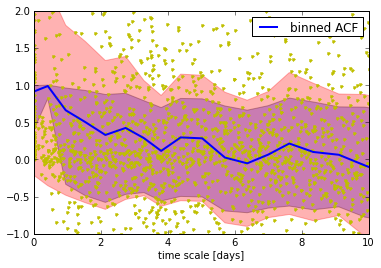For short times (< 2 days) the ACF is clearly outside the box where we would expect to find the ACF of a lightcurve consisting of white noise only. However, for larger times scales, we have to be careful, because the value of the ACF is actually not much larger than the ACF expected for white noise.

### Suggestion for a more robust measure of autocorrelation¶

The cumulative sum of a function is always more robust than the function itself, because noise tends to cancel out. Thus, I can use the small binning I want.

In :
for scale in [0.1, 0.5, 1.0]:
timebins = np.arange(0, np.max(dt), scale)
autocorr, n_in_bin = lc.slotting(timebins, dt, dm)
plt.plot(timebins + scale, scale * autocorr.cumsum(), lw = 2., label = 'bin width = ' + str(scale))
plt.xlim([0,10])
plt.ylim([0,3])
plt.xlabel('time scale [days]')
plt.ylabel('cumulative sum')
plt.plot(plt.xlim(), [1.75,1.75],'k', label = 'proposed cut')
plt.legend(loc = 'lower right')

Out:
<matplotlib.legend.Legend at 0x9d09110>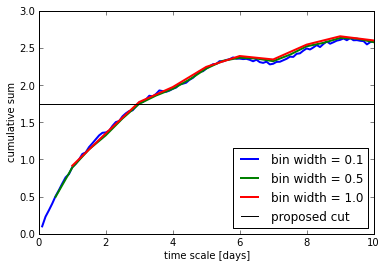In :
scale = .1
timebins = np.arange(0, np.max(dt), scale)
autocorr, n_in_bin = lc.slotting(timebins, dt, dm)
autocorr[~np.isfinite(autocorr)] = 0
plt.plot(timebins + scale, scale*autocorr.cumsum(), lw = 2.)
#plt.plot(timebins, n_in_bin)
plt.xlim([0,10])
plt.ylim([0,3])
#plt.xlabel('time scale [days]')
#plt.ylabel('cumulative sum')
#plt.plot(plt.xlim(), [1.75,1.75],'k', label = 'proposed cut')
#plt.legend(loc = 'lower right')

Out:
(0, 3)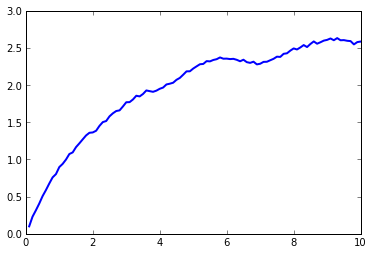In :
ind = np.argsort(dt)
plt.plot(dt[ind], (dm[ind]).cumsum() * dt[ind] / np.arange(len(dt)))
plt.xlim([0,10])
plt.ylim([0,3])

Out:
(0, 3)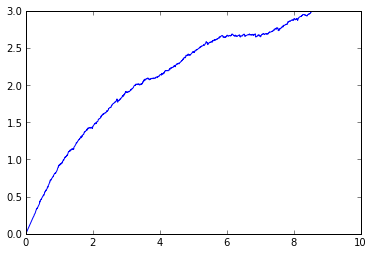Now, the value depends only weakly on the binning chosen. So, I can use the small binning to achieve a good resolution (so that different datasets have a chance to give different results). On the flip side, if I measure "where does the cumulative sum of the autocorrelation function hit 1.75", there is no direct relation between the measured value and the underlying time scale. Only to say that "more correlated" lightcurves will reach 1.75 after short times and "less correlated" lightcurves with do so later (because their autocorrelation function drops faster). Uncorrelated lightcurves will never reach a cumulative some of 1.75.

The value of 1.75 however is totally arbitrary. It's chosen as "must be larger than 1.0 and must be so small that most lightcurves actually make it there".

#### How would the cumulative sum look like for different uncorrelated functions?¶

In :
def whitenoise(t):
return np.random.normal(size = len(t))

def trendwhitenoise(t, slope = 1.):
return (t-np.min(t)) * slope + whitenoise(t)

def periodwhitenoise(t, a = 1, omega = 1.):
return a * np.sin(omega * t) + whitenoise(t)

In :
scale = 0.1
tq1 = t[t < 55400]
timebins = np.arange(0, np.max(tq1), scale)
fig = plt.figure(figsize = (20,4))

for i in range(20):
mq1 = periodwhitenoise(tq1, a = 1.5)
m_norm = lc.normalize(mq1)
ax1.plot(tq1, m_norm)
dts, dms = lc.delta_corr_points(tq1, m_norm, m_norm)
timebins = np.arange(0, np.max(dts), scale)
autocorr, n_in_bin = lc.slotting(timebins, dts, dms)
ax3.plot(timebins + scale, scale * autocorr.cumsum(), label = 'bin width = ' + str(scale))
ax2.plot(timebins, autocorr, label = 'bin width = ' + str(scale))
dtsf, dmsf = lc.corr_points(tq1, m_norm, m_norm)
timebins, dsf = lc.discrete_struc_func(tq1, mq1-np.mean(mq1), scale = scale)
ax4.plot(timebins, dsf)
ax2.set_xlim([0,10])
ax2.set_ylim([-1,2])
ax2.set_xlabel('time scale [days]')
ax2.barh(-(1./len(tq1) + 3./np.sqrt(len(tq1))), 10., height = 2. * 3 / np.sqrt(len(tq1)), color = 'y')
ax2.set_title('ACF')
ax1.set_xlabel('MJD')
ax1.set_title('Lightcurves')
ax3.set_title('Cumulative sum of ACF')
ax3.set_xlim([0,10])
ax3.set_ylim([0,3])
ax3.set_xlabel('time scale [days]')
ax3.set_ylabel('cumulative sum')
ax3.plot(ax3.get_xlim(), [1.75,1.75],'k', label = 'proposed cut')
ax4.set_xlim([0,10])
ax4.set_ylim([0,6.])
ax4.set_title('Structure function')

Out:
<matplotlib.text.Text at 0x679d2d0>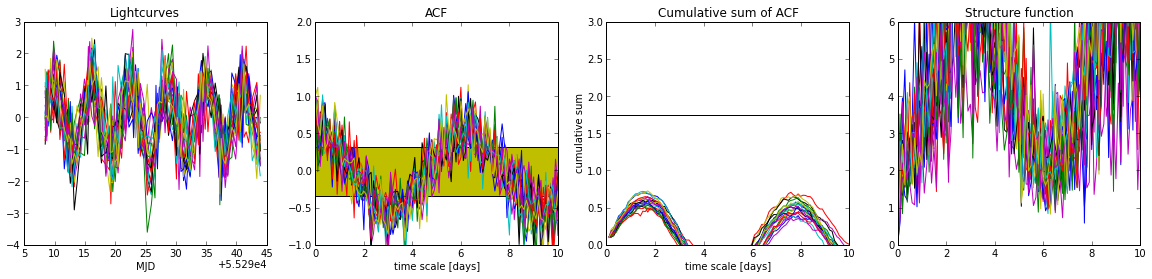### Scatterplots¶

Another way to show that a correlation exists on time scale of a few days is to make a scatter plot of $m_i$ vs. $m_j$ for all magnitudes $m$, where the time difference $\delta t = t_j - t_i\$ is in a certain range. If there were no correlation on this scale, then we expect a point could. If these values are correlated, i.e. high $m$ is most likely followed by another high $m$ at a time $\delta t$ later, then we should see a kind of linear correlation (Percival & Walden, 1993).

In :
ind = (dt > 2.) & (dt < 3.)

In :
dt_2d, m_2d = lc.corr_points(t,m,m)

In :
ind = (dt_2d > 2 ) & (dt_2d < 4)
plt.scatter(m_2d[ind,0], m_2d[ind,1])
ax = plt.gca()
ax.invert_yaxis()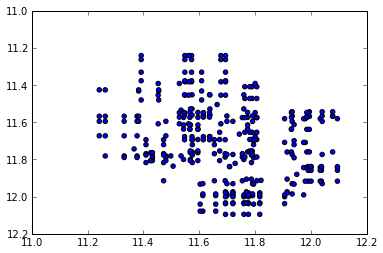In :
print "Probability of no correlation in the data", scipy.stats.pearsonr(m_2d[ind,0], m_2d[ind,1])

Probability of no correlation in the data 8.67294452672e-08


Here, a look on the plot shows a correlation and this is statistically firmed up by a Pearson rank test, that indicates a negligible chance to obtain such a distribution from a random sample ( = a non-correlated lightcurve).

### Discrete structure function (DSF)¶

In :
for scale in [0.1, 0.5, 1.0]:
timebins, dsf = lc.discrete_struc_func(t, m, scale = scale)
plt.plot(timebins, dsf, lw = 2., label = 'bin width = ' + str(scale))
plt.xlim([0,10])
plt.ylim([0,.1])
plt.xlabel('time scale [days]')
plt.legend(loc = 'lower right')

Out:
<matplotlib.legend.Legend at 0xb729950>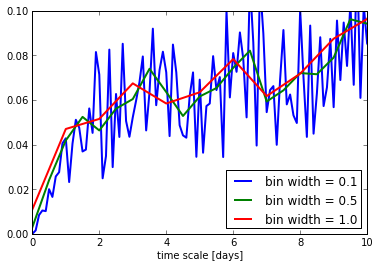#### For randomn values, how does the DSF look?¶

Let's assume we look at a lightcurve of white noise, where each measurement is drawn independently form a normal distribution with $\mu=0$ and $\sigma=1$. Then, let us see which values the discrete crete structure function takes. For our real application we want to bin all available points according to their $\delta t$. So, we next look at the distribtion of values for the DSF that we expect when each $\delta t$ bin has a certain number of points in it. We average over each bin and ask what the distribution of average values is for a white noise lightcurve.

In :
X = np.random.normal(size = (1000000,2))
dsf_points = (X[:,0]-X[:,1])**2.
fig = plt.figure(figsize = (20,4))
res = ax1.hist(dsf_points, bins = 100, normed = True)
ax1.set_title('Distribution of $(X_1-X_2)^2$')
res = ax2.hist(dsf_points.reshape((-1, 10)).mean(axis=1), bins=100, normed = True)
ax2.set_title('Distribution of DSF (10 points oer bin)')

Out:
<matplotlib.text.Text at 0x6040050>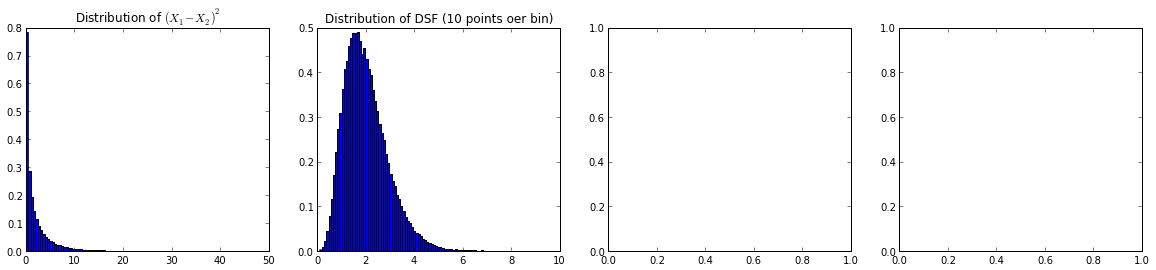## Rebinned lightcurve¶

I'll use the data form the first epoch only and rebin it to 0.1 days using a linear piecewise interpolation. Clearly, that will introduce a new autocorrelation on the scale of $\delta t$, so we need to ignore anything shorter than about 1 day. I show the interpolated lightcurve in the next plot below. The red squares are the original datapoints, the blue dots the new, interpolated values.

In :
scale = .1
trebin = np.arange(np.min(t), np.max(t[t < 55340]), scale)
mrebin = np.interp(trebin, t, m)
m_rnorm = lc.normalize(mrebin)

In :
plt.plot(trebin, mrebin,'.', label = 'rebinned')
plt.plot(t, m,'rs', label = 'original')
plt.xlim([55290, 55340])
ax = plt.gca()
ax.invert_yaxis()
plt.ylabel('[3.6]')
plt.xlabel('MJD')
plt.legend()

Out:
<matplotlib.legend.Legend at 0x149c2890>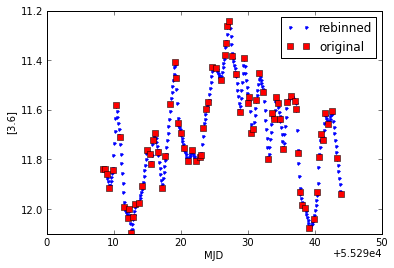In :
import statsmodels.tsa.stattools as tsatools
acf = tsatools.acf(m_rnorm, nlags = 30./scale)
plt.plot(np.arange(len(acf)) * scale, acf)
plt.xlabel('time scale [days]')
plt.ylabel('autocorrelation coefficient')
plt.title('Autocorrelation function')
plt.grid()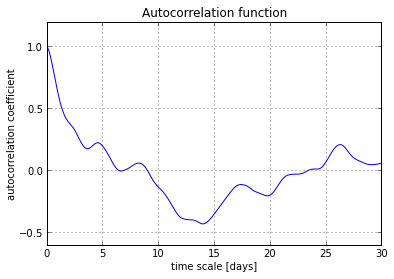The plot shows the auto correlation function (ACF) of the interpolated lightcurve. The comparison with the scatter plot above shows that this gives essentially the same answer, everything it just much smoother. (Maybe that is what Maria did to her lightcurves to calculate the values she defined? - I did not check her IDL implementation). So, I can use Maria's definition on rebinned lightcurves. First of all, that's good news. Also (not shown here), the function is basically independent of the bin size chosen for the rebinned lightcurve.

However, the first time the function falls below 0.5 is here (and in other examples I looked at) at just around a day. Given that the $\delta t$ between some measurements is of similar order, the "time where the autocorrelation function falls below 0.5" is to some degree influenced by the interpolation used. The lightcurve is interpolated piecewise linearly, so it's no surprise that all elements interpolated onto the same piece are well correlated. To get out of this regime, I will take the value where the autocorrelation function falls below 0.25 for the first time. I don't see a good reason to choose any particular number over the other, the choice is arbitrary and (apart from the problem of measuring a time scale that is still influenced by the interpolations scheme) 1/2, 1/4, 1/e or 0.123456789 are all equally valid choices.

Trying to go a little further, I now calculate the "partial autocorrelation function". Peaks in this function should mark the position of the most important time scales that are responsible for the autocorrelation.

In :
pacf = tsatools.pacf(m_rnorm, nlags = np.int(12./scale), method = 'ols')

In :
plt.plot(np.arange(len(pacf)) * scale, pacf)
plt.grid()
plt.xlabel('timescale [days]')
plt.ylabel('coeffictions of paritial autocorrelation function')
plt.title('Partial autocorrelation function')

Out:
<matplotlib.text.Text at 0x1e657110>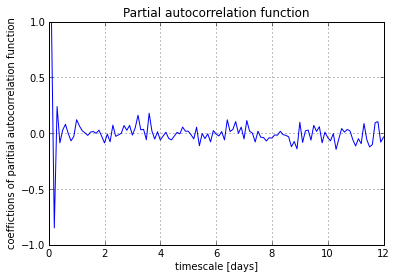I don't see any time scale > 1 day really sticking out here. That could mean that there is a distribution of important time scales or that the signal in the partial autocorrelation function is just totally dominated by the correlations on short time scales that are artifacts of the interpolation scheme.

### Autoregressive (AR) and autoregressive moving-average (ARMA) models¶

#### AR models¶

AR models describe a discrete lightcurve with a stochastic model, where the value of each element of the lightcurve depends on one or more directly preceding elements and some random Gaussian noise. Wikipedia has a reasonable introduction to this class of models and lists the definition. These models are apparently very common in finance to describe (and predict) stock values. Anyway, I just want to explore here how far I can get using those models. When I show "fits" below, remember that these are not "fits" in a $\chi^2$ sense. Since each point of the model has a random contribution, different runs using the same model parameters will give different curves and different $\chi^2$.

I'll repeat the code for rebinning the lightcurve here to experiment with other bin sizes than in the last section.

In :
scale = .5
trebin = np.arange(np.min(t), np.max(t[t < 55340]), scale)
mrebin = np.interp(trebin, t, m)
m_rnorm = lc.normalize(mrebin)

In :
import statsmodels.tsa.ar_model as ar
modar = ar.AR(m_rnorm)
resar = modar.fit(1)


Select the best order for a model, i.e. the number of preceding elements that a new elements depends on. Clearly, a higher order will make a better fit, but also require more parameters. The Akaike information criterion (AIC) is one way to judge the best order. Other criteria are discussed in the literature. I tried a few. The "best" order for a model changes a little with the criterion used, but not much.

In :
modar.select_order(20, 'aic')

Out:
9
In :
resar = modar.fit(2)

In :
print 'fit params: ', resar.params, '\nsigma of noise: ', resar.sigma2, '\nAIC:', resar.aic

fit params:  [-0.00142603  1.27715705 -0.4650391 ]
sigma of noise:  0.192558110144
AIC: -1.56040077819

In :
plt.plot(trebin[resar.k_ar:], resar.fittedvalues)
plt.plot(trebin, m_rnorm)
plt.xlabel('time [days]')
plt.ylabel('$\\delta m$ [normalized]')

Out:
<matplotlib.text.Text at 0xb5efbd0>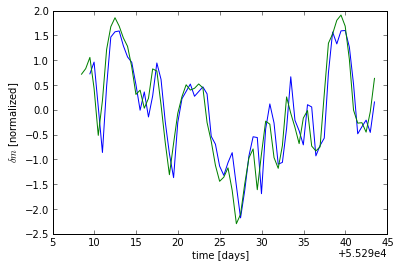Clearly, even a very simple model of order 2 (much less than the best order estimated) can produce lightcurves that are qualitatively very, very similar to what we observe. Note that order 2 does not imply that the relevant time scales are very short. Even for order 1 element $x_i$ of the time series depends on $x_{i-1}$ and that in turn on $x_{i-2}$ and that on $x_{i-3}$ etc. so that each element influences the entire future of the lightcurve, the fitted coefficients just describe how fast this influence decays.

Independent of the actual values of the parameters, this means that the lightcurves we observe can be well described by an autoregressive model with some stochastic randomness (see the value for $\sigma$ noise printed above). Note that this $\sigma$ is not the same as the measurement error, instead the $\sigma$ is a stochastic contribution to the true value of the magnitude.

Unfortunately, the value of $\sigma$ depends strongly on the binning of the resampled lightcurve. Imagine looking at a part of the new lightcurve that contains more than two values sampled from the same piece (in a timestep in the original lightcurve that was large). There, the third and every later point sampled from the same piece are exactly determined by the two preceding points.

Thus, we should probably use a relatively rough resampling where even for the longest time steps only one or two point of the new lightcurve are sampled from the same piece. Unfortunately, resampling to e.g. regular intervals of 1 day reduces the lightcurve from about 105 datapoints to only 35 datapoints - If we throw out that much information, is there actually enough left to learn something we did not see in the autocorrelation function? On the other hand, most YSOVAR sources in the field are less densely sampled and if I rebin them all to 1 / day then at least I get an apples-to-apples comparison.

For a binning that is large enough the value of $\sigma$ is slightly more useful. For a binning of 0.5 days I here find $\sigma = 0.2\$ (in the normalized lightcurve).

#### ARMA models¶

In the AR part, ARMA models introduce a dependence on the value of the stochastic noise chosen in one or more of the preceding values. I chose an ARMA model with AR order 2 and MA order 1.

In :
import statsmodels.tsa.arima_model as arima
model = arima.ARMA(m_rnorm)

In :
res = model.fit((2,1))

In :
plt.plot(trebin[:res.nobs], res.fittedvalues)
plt.plot(trebin,m_rnorm)
plt.xlabel('time [days]')
plt.ylabel('$\\delta m$ [normalized]')

Out:
<matplotlib.text.Text at 0x55d5e10>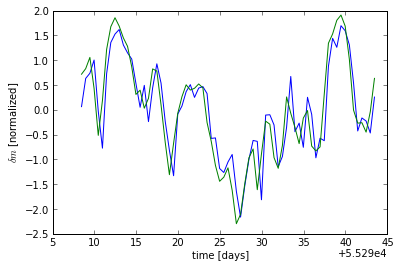In :
print 'fit params: ', res.params, '\nsigma of noise: ', res.sigma2, '\nAIC: ', res.aic

fit params:  [ 0.06412878  0.94343754 -0.17856475  0.46228946]
sigma of noise:  0.174804661209
AIC:  89.7646295574


Comparing ARMA (2,0) (which essentially is an AR model - not plotted again) and ARMA(2,1) I see very little changes in the fitted parameters. I first suspected that this result might be an artifact of the interpolation scheme, but I played around with the scaling of the rebinned lightcurve and, while many things change, the difference between AR and ARMA (for the same degree of AR) stays small.

The AIC for ARMA(2,0) and ARMA(2,1) is not very different, I don't know why the absolute values of the AR(2) model shown above is much higher, although the fitted values are almost the same. I suspect that that's a bug in the implementation of statsmodels.

#### Interpretation¶

In finance, one interpretation of stock market curves (as far as I understand it) is that there is some long-term trend that represents the long-term economic value of the company. The AR part would be the herd of investors, whose decisions depend on the value the of the stock in the past, but most strongly in the last few days and the MA part would be outside shocking (news about the unemployment rate etc.) that have short term effect on the price, but the influence decays fast with time.

How do I interpret these models for our YSOVAR lightcurves? I don't really know. The fact that ARMA and AR models seem to describe the data almost equally well tell me that the MA part (quickly decaying random processes) is not important. As for numbers to compare different sources, there is not too much to gain: If the lightcurve is well described by an autoregressive model, then most of the information is already contained in the autocorrelation function. The shape of the ACF already tells us that the lightcurves are stocastic, but not random and from the shape of the ACF we have a fairly obvious way to somewhat quantify the strength of the correlation.

Caveat: All the analysis above is done for a single exemplary lightcurve.

### Conclusion from looking at the exemplary lightcurve¶

After going through all this exercise I conclude that the measures Maria suggested to quantify the timing behavior of the YSOVAR lightcurves are already pretty good, as long as the lightcurve is resampled on a fine grid before the autocorrelation function is calculated. I suggest to lower the threshold value for the "coherence time" to leave the time regime that is still strongly influenced by the choice of the resampling and I suggest that the cumulative sum of the unbinned ACF reaching 1.75 can be used to characterize the ACF without binning.

## The sample of lightcurves in L1688¶

Now, I need to run an analysis similar to the above on all lightcurves that I have for L1688 and calculate the numbers Maria used with the modifications I suggested. To this end I coded them into our module pYSOVAR.lightcurves that I already imported above.

I calculate the numbers for all IRAC1 lightcurves in L1688 with more than 50 entries.

In :
acf = np.zeros((len(data), 4))
for i, d in enumerate(data):
if 't36' in d.keys() and len(d['t36']) >50:
ind = d['t36'] < 55340
coherence_time, autocorr_time, autocorr_val, cumsumtime = lc.describe_autocorr(d['t36'][ind], d['m36'][ind], \
autocorr_scale = 0.25, autosum_limit = 2.5)
else:
coherence_time, autocorr_time, autocorr_val, cumsumtime = (np.nan, np.nan, np.nan, np.nan)
acf[i,0] = coherence_time
acf[i,1] = autocorr_time
acf[i,2] = autocorr_val
acf[i,3] = cumsumtime

In :
plt.plot(acf[:,0], acf[:,3], 'bs')
plt.ylim(0,10)
plt.xlabel('coherence time')
plt.ylabel('time for cumulative ACF to reach 1.75')

Out:
<matplotlib.text.Text at 0x55d8a10>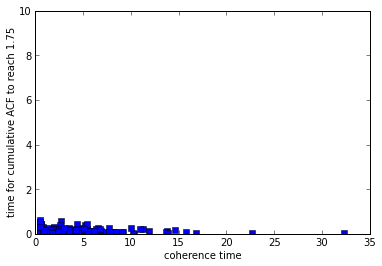Lightcurves with long coherence times have autocorrelations that drop off only slowly and the cumulative sum reaches 1.75 after a short time. On the other hand, lightcurves with a short coherence time need a long time until their cumulative sums reach 1.75 by random walk. Both measures are clearly correlated for short coherence times.

In :
plt.plot(acf[:,0], acf[:,1], 'bs')
plt.xlabel('coherence time')
plt.ylabel('position of first peak in ACF')
#plt.ylim(0,40)

Out:
<matplotlib.text.Text at 0x5cac610>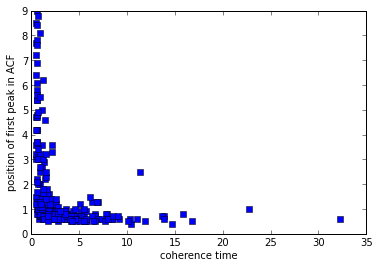Lightcurves with a larger coherence time also have the position of the first peak at larger values. That is actually expected, given that the first peak can only occur after the ACF dropped below zero. I am not quite sure how much information the peak position actually holds. Since the data only spans a little more than a month, all very long numbers are suspicious and for short coherence times, the position of the first peak (see plot below) follows a quite well defined distribution, that is somewhat reminiscent of a binomial distribution. I do not see any significant peak that sticks out as the "typical" time scale.

In :
plt.hist(acf[:,1], range= [0,10])

Out:
(array([321,  62,  14,  11,   9,   5,   4,   4,   5,   0]),
array([  0.,   1.,   2.,   3.,   4.,   5.,   6.,   7.,   8.,   9.,  10.]),
<a list of 10 Patch objects>)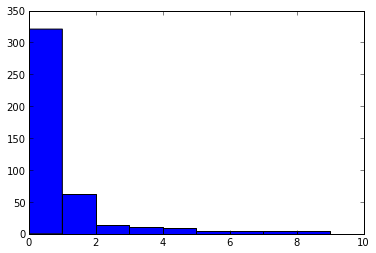In :
plt.plot(acf[:,1], acf[:,2], 'bs')
plt.ylabel('Height of first peak')
plt.xlabel('position of first peak [days]')

Out:
<matplotlib.text.Text at 0x57b0390>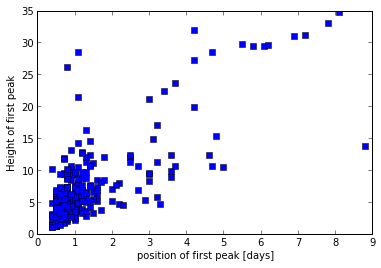Only very few lightcurves have high first peaks above, let's say 0.3 or so. For the peaks occurring at very short time scales, we need to be suspicious. Given that the position of the first peak will always be larger than the coherence time scale, the coherence time scale for those lightcurves must be very small. What most likely happens here, is that the lightcurves are noisy, and fluctuate very strongly due to noise so that they have, e.g. a negative value already at 0.5 days. For peaks that occur at large time scales, we again need to be suspicious. Since the time span covered by the observations is only a little more than month, very few data point to into those peaks.

In :
ar_models = np.zeros((len(data), 5))
for i, d in enumerate(data):
if 't36' in d.keys() and len(d['t36']) >50:
ind = d['t36'] < 55340
params, sigma2, aic = lc.ARmodel(d['t36'][ind], d['m36'][ind])
else:
params, sigma2, aic = (np.nan, np.nan, np.nan)
ar_models[i,0:3] = params
ar_models[i,3] = sigma2
ar_models[i,4] = aic

In :
plt.plot(acf[:,0], ar_models[:,3], 'bs')
plt.xlabel('ACF coherence time [days]')
plt.ylabel('$\\sigma_2$ after fitting AR(2)')
plt.title('Compare ACF and AR models')

Out:
<matplotlib.text.Text at 0x5b7dd50>